##### Physics I Workbook For Dummies with Online PracticeKinetic friction is usually less than the force of friction you need to overcome static friction. Kinetic friction occurs when an object is already in motion.

Static friction is the force that must be overcome to get something to move, and it’s usually larger than kinetic friction, the force that pushes against a moving object. The equation for kinetic friction is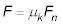In order to keep an object moving at a constant velocity, a force must be applied to that object which exactly balances the force from kinetic friction.

## Sample question

1. Suppose you have a 5.0 kg block of ice, and it takes 5 N to keep it moving across the floor at a constant velocity. What is the coefficient of kinetic friction,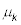1. The force of kinetic friction is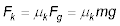2. Solve for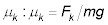3. Plug in the numbers: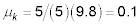## Practice questions

1. You’re cross-country skiing and need 20 N of force to maintain your velocity. If you have a mass of 70 kg, what is the coefficient of kinetic friction:2. You’re skating and need 17 N to maintain your velocity. If you have a mass of 80 kg, what is the coefficient of kinetic friction:Following are answers to the practice questions:

1. 0.03

1. Solve forThe force due to friction is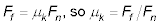2. The force due to friction that you have to overcome, Ff, is 20 N, and the normal force, Fn, is 70g = 690 N.

3. Use the equation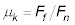to get the coefficient of kinetic friction: 20/690 = 0.03.

2. 0.02

1. Solve forThe force due to friction is2. The force due to friction that you have to overcome, Ff, is 17 N, and the normal force, Fn, is 80g = 780 N.

3. Use the equationto get the coefficient of kinetic friction: 17/780 = 0.02.